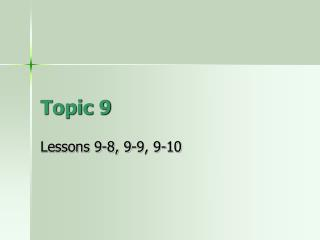DownloadDownload PresentationTopic 9

# Topic 9

Télécharger la présentation## Topic 9

- - - - - - - - - - - - - - - - - - - - - - - - - - - E N D - - - - - - - - - - - - - - - - - - - - - - - - - - -
##### Presentation Transcript

1. Topic 9 Lessons 9-8, 9-9, 9-10

2. POD A group of 36 students goes on a school trip. Of all the students on the trip, 18 are in third grade. What is 18/36 in simplest form? • A. 1/3 • B. 4/12 • C. 8/24 • D. 1/2

3. POD A group of 36 students goes on a school trip. Of all the students on the trip, 18 are in third grade. What is 18/36 in simplest form? • A. 1/3 • B. 4/12 • C. 8/24 • D. 1/2

4. How can you write a fraction as a decimal? • The word name for 3/10 is three tenths. • What would three tenths look like as a decimal? • 0.3 • So, 3/10 = 0.3

5. Think about money… • You know that \$0.13 means 13 cents. • What fraction of a dollar does this decimal represent? • If there are 100 cents in a dollar… • Then 13 cents is… • 13/100 of a dollar

6. You try some • 0.15 = 15/100 • 0.23 = ? • 0.31 = ? • 45/100 = 0.45 • 63/100 = ? • 71/100 = ?

7. You try some • 0.15 = 15/100 • 0.23 = 23/100 • 0.31 = 31/100 • 45/100 = 0.45 • 63/100 = 0.63 • 71/100 = 0.71 These are all hundredths

8. Now try these • 4/10 = 0.4 • 6/10 = ? • 2/10 = ? • 0.9 = 9/10 • 0.7 = ? • 1/10 = ?

9. Now try these • 4/10 = 0.4 • 6/10 = 0.6 • 2/10 = 0.2 • 0.9 = 9/10 • 0.7 = 7/10 • 1/10 = 1/10 These are all what? Tenths

10. Now add whole numbers • 9 3/10 = 9.3 • 3 23/100 = 3.23 • 4 5/10 = ? • 6 43/100 = ?

11. Now add whole numbers • 9 3/10 = 9.3 • 3 23/100 = 3.23 • 4 5/10 = 4.5 • 6 43/100 = 6.43

12. Textbook page 238-239 • Lets look at #1-4 • On your own do #12-27

13. Thousandths • What would 0.454 look like as a fraction? • Think about how you would say this number in word form… • Four hundred fifty-four thousandths • So… • 454/1000

14. Try these • 0.647 = 647/1000 • 0.342 = ? • 0.876 = ? • 142/1000 = 0.142 • 272/1000 = ? • 943/1000 = ?

15. Try these • 0.647 = 647/1000 • 0.342 = 342/1000 • 0.876 = 876/1000 • 142/1000 = 0.142 • 272/1000 = 0.272 • 943/1000 = 0.943

16. Textbook page 242 • #1-8 together • Homework: page 242 #11-26

17. POD Quick check • What is 94/1000 as a decimal? • A. 0.904 • B. 0.094 • C. 0.0904 • D. 0.0094 • 1. What is 0.008 as a fraction? • 9/1 • 9/10 • 9/100 • 9/1000 • A kitten weighed 0.128 kilograms when it was born. • What is 0.128 as a fraction? • 128/1000 • 128/100 • 128/10 • 128/1

18. Number line on the board

19. Fractions and decimals on a number line • Where do you think these two should go? 0.5 and 1/10 0.4 and 7/10 1/2 0 0.5 1 0.4 7/10 1/10

20. Workbook pages 157-158 • Or textbook pages 244-245 #1-13 • Homework/class work: • quick check 9-11

21. Tomorrow Review for TEST